# Creating FFTs with FFTrees()

#### 2018-09-15

The `FFTrees()` function is at the heart of the `FFTrees` package. The function takes a training dataset as an argument, and generates several fast-and-frugal trees which attempt to classify cases into one of two classes (True or False) based on cues (aka., features).

## Example: heartdisease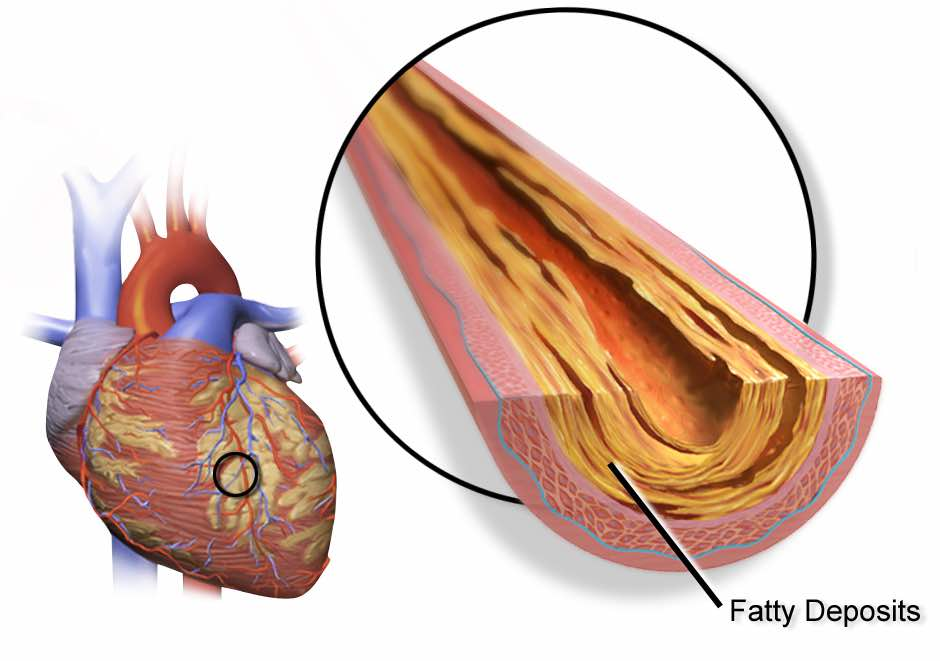We’ll create FFTrees for heartdisease diagnosis data. The full dataset is stored as `heartdisease`. For modelling purposes, I’ve split the data into a training (`heart.train`), and test (`heart.test`) dataframe. Here’s how they look:

``````# Training data
``````## # A tibble: 6 x 14
##   diagnosis   age   sex cp    trestbps  chol   fbs restecg   thalach exang
##   <lgl>     <dbl> <dbl> <chr>    <dbl> <dbl> <dbl> <chr>       <dbl> <dbl>
## 1 FALSE        63     1 ta         145   233     1 hypertro…     150     0
## 2 TRUE         67     1 a          160   286     0 hypertro…     108     1
## 3 TRUE         67     1 a          120   229     0 hypertro…     129     1
## 4 FALSE        37     1 np         130   250     0 normal        187     0
## 5 FALSE        41     0 aa         130   204     0 hypertro…     172     0
## 6 FALSE        56     1 aa         120   236     0 normal        178     0
## # ... with 4 more variables: oldpeak <dbl>, slope <chr>, ca <dbl>,
## #   thal <chr>``````
``````# Test data
``````## # A tibble: 6 x 14
##   diagnosis   age   sex cp    trestbps  chol   fbs restecg   thalach exang
##   <lgl>     <dbl> <dbl> <chr>    <dbl> <dbl> <dbl> <chr>       <dbl> <dbl>
## 1 FALSE        63     1 ta         145   233     1 hypertro…     150     0
## 2 TRUE         67     1 a          160   286     0 hypertro…     108     1
## 3 TRUE         67     1 a          120   229     0 hypertro…     129     1
## 4 FALSE        37     1 np         130   250     0 normal        187     0
## 5 FALSE        41     0 aa         130   204     0 hypertro…     172     0
## 6 FALSE        56     1 aa         120   236     0 normal        178     0
## # ... with 4 more variables: oldpeak <dbl>, slope <chr>, ca <dbl>,
## #   thal <chr>``````

The critical dependent variable is `diagnosis` which indicates whether a patient has heart disease (`diagnosis = 1`) or not (`diagnosis = 0`). The other variables in the dataset (e.g.; sex, age, and several biological measurements) will be used as predictors (aka., cues).

## Create trees with `FFTrees()`

We will train the FFTs on `heart.train`, and test their prediction performance in `heart.test`. Note that you can also automate the training / test split using the `train.p` argument in `FFTrees()`. This will randomly split `train.p`% of the original data into a training set.

To create a set of FFTs, use `FFTrees()`. We’ll create a new FFTrees object called `heart.fft` using the `FFTrees()` function. We’ll specify `diagnosis` as the (binary) dependent variable, and include all independent variables with `formula = diagnosis ~ .`

``````# Create an FFTrees object called heart.fft predicting diagnosis
heart.fft <- FFTrees(formula = diagnosis ~.,
data = heart.train,
data.test = heart.test)``````
• If we wanted to only consider specific variables, like sex and age, for the trees we could do this by specifying `formula = diagnosis ~ age + sex`

## Elements of an FFTrees object

`FFTrees()` returns an object with the FFTrees class. There are many elements in an FFTrees object, here are their names:

``````# Print the names of the elements of an FFTrees object
names(heart.fft)``````
``````##   "formula"          "data.desc"        "cue.accuracies"
##   "tree.definitions" "tree.stats"       "cost"
##   "level.stats"      "decision"         "levelout"
##  "tree.max"         "inwords"          "params"
##  "comp"             "data"``````
• `formula`: The formula used to create the FFTrees object.
• `data.desc`: Basic information about the datasets.
• `cue.accuracies`: Thresholds and marginal accuracies for each cue.
• `tree.definitions`: Definitions of all trees in the object.
• `tree.stats`: Classification statistics for all trees (tree definitions are also included here).
• `level.stats`: Cumulative classification statistics for each level of each tree.
• `decision`: Classification decisions for each case (row) for each tree (column).
• `levelout`: The level at which each case (row) is classified for each tree (column).
• `auc`: Area under the curve statistics
• `params`: Parameters used in tree construction
• `comp`: Models and statistics for alternative classification algorithms.

You can view basic information about the FFTrees object by printing its name. The default tree construction algorithm `ifan` creates multiple trees with different exit structures. When printing an FFTrees object, you will see information about the tree with the highest value of the `goal` statistic. By default, `goal` is weighed accuracy `wacc`:

``````# Print the object, with details about the tree with the best training wacc values
heart.fft``````
``````## FFT 1 (of 7) predicts diagnosis using 3 cues: {thal, cp, ca}
##
##  If thal = {rd,fd}, decide True.
##  If cp != {a}, decide False.
##  If ca <= 0, decide False, otherwise, decide True.
##
##                    train   test
## cases       .n    150.00 153.00
## hits        .hi    54.00  64.00
## misses      .mi    12.00   9.00
## false al    .fa    18.00  19.00
## corr rej    .cr    66.00  61.00
## speed       .mcu    1.74   1.73
## frugality   .pci    0.88   0.88
## cost        .cost   0.20   0.18
## accuracy    .acc    0.80   0.82
## balanced    .bacc   0.80   0.82
## sensitivity .sens   0.82   0.88
## specificity .spec   0.79   0.76
##
## pars: algorithm = 'ifan', goal = 'wacc', goal.chase = 'wacc', sens.w = 0.5, max.levels = 4``````

Here is a description of each statistic:

statistic long name definition
`n` N Number of cases
`mcu` Mean cues used On average, how many cues were needed to classify cases? In other words, what percent of the available information was used on average.
`pci` Percent cues ignored The percent of data that was ignored when classifying cases with a given tree. This is identical to the `mcu / cues.n`, where `cues.n` is the total number of cues in the data.
`sens` Sensitivity The percentage of true positive cases correctly classified.
`spec` Specificity The percentage of true negative cases correctly classified.
`acc` Accuracy The percentage of cases that were correctly classified.
`wacc` Weighted Accuracy Weighted average of sensitivity and specificity, where sensitivity is weighted by `sens.w` (by default, `sens.w = .5`)

### Cue accuracy statistics: cue.accuracies

Each tree has a decision threshold for each cue (regardless of whether or not it is actually used in the tree) that maximizes the `goal` value of that cue when it is applied to the entire training dataset. You can obtain cue accuracy statistics using the calculated decision thresholds from the `cue.accuracies` list. If the object has test data, you can see the marginal cue accuracies in the test dataset (using the thresholds calculated from the training data):

``````# Show decision thresholds and marginal classification training accuracies for each cue
heart.fft\$cue.accuracies\$train``````
``````##         cue     class            threshold direction   n hi fa mi cr  sens
## 1       age   numeric                   54         > 150 47 31 19 53 0.712
## 2       sex   numeric                    0         > 150 53 48 13 36 0.803
## 3        cp character                    a         = 150 48 18 18 66 0.727
## 4  trestbps   numeric                  138         > 150 26 21 40 63 0.394
## 5      chol   numeric                  223         > 150 49 51 17 33 0.742
## 6       fbs   numeric                    0         > 150 10  9 56 75 0.152
## 7   restecg character hypertrophy,abnormal         = 150 40 34 26 50 0.606
## 8   thalach   numeric                  156        <= 150 47 31 19 53 0.712
## 9     exang   numeric                    0         > 150 31 14 35 70 0.470
## 10  oldpeak   numeric                  0.9         > 150 41 21 25 63 0.621
## 11    slope character            flat,down         = 150 45 27 21 57 0.682
## 12       ca   numeric                    0         > 150 47 19 19 65 0.712
## 13     thal character                rd,fd         = 150 47 16 19 68 0.712
##     spec  bacc   acc  wacc costout   cost costcue
## 1  0.631 0.672 0.667 0.672  -0.333 -0.333       0
## 2  0.429 0.616 0.593 0.616  -0.407 -0.407       0
## 3  0.786 0.756 0.760 0.756  -0.240 -0.240       0
## 4  0.750 0.572 0.593 0.572  -0.407 -0.407       0
## 5  0.393 0.568 0.547 0.568  -0.453 -0.453       0
## 6  0.893 0.522 0.567 0.522  -0.433 -0.433       0
## 7  0.595 0.601 0.600 0.601  -0.400 -0.400       0
## 8  0.631 0.672 0.667 0.672  -0.333 -0.333       0
## 9  0.833 0.652 0.673 0.652  -0.327 -0.327       0
## 10 0.750 0.686 0.693 0.686  -0.307 -0.307       0
## 11 0.679 0.680 0.680 0.680  -0.320 -0.320       0
## 12 0.774 0.743 0.747 0.743  -0.253 -0.253       0
## 13 0.810 0.761 0.767 0.761  -0.233 -0.233       0``````

You can also view the cue accuracies in an ROC plot with `plot()` combined with the `what = "cues"` argument. This will show the sensitivities and specificities for each cue, with the top 5 cues highlighted.

``````# Visualize individual cue accuracies
plot(heart.fft,
main = "Heartdisease Cue Accuracy",
what = "cues")``````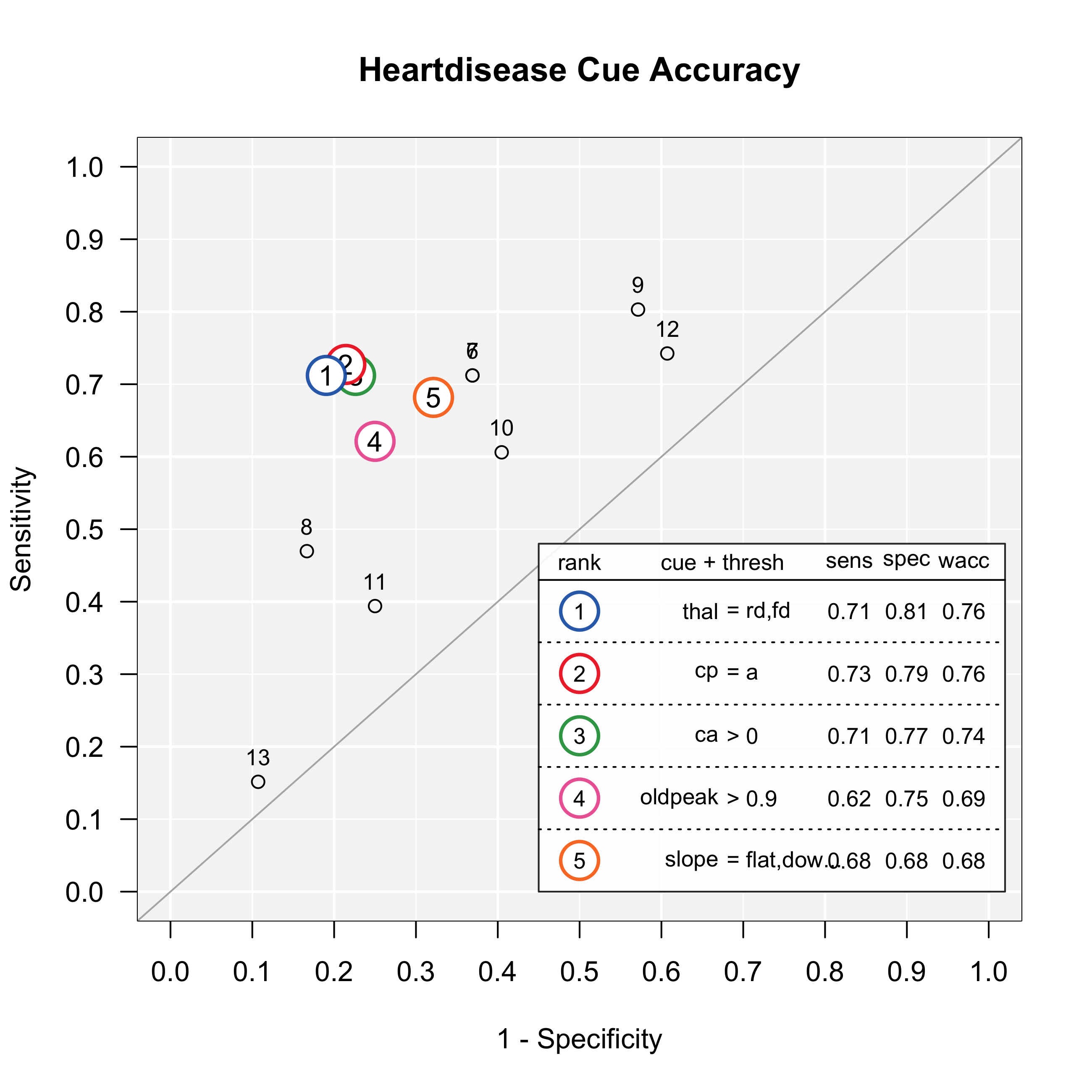### Tree definitions

The `tree.definitions` dataframe contains definitions (cues, classes, exits, thresholds, and directions) of all trees in the object. The combination of these 5 pieces of information (as well as their order), define how a tree makes decisions.

``````# Print the definitions of all trees
heart.fft\$tree.definitions``````
``````##   tree nodes classes               cues directions    thresholds     exits
## 1    1     3   c;c;n         thal;cp;ca      =;=;>     rd,fd;a;0   1;0;0.5
## 2    2     4 c;c;n;n thal;cp;ca;oldpeak    =;=;>;> rd,fd;a;0;0.9 1;0;1;0.5
## 3    3     3   c;c;n         thal;cp;ca      =;=;>     rd,fd;a;0   0;1;0.5
## 4    4     4 c;c;n;n thal;cp;ca;oldpeak    =;=;>;> rd,fd;a;0;0.9 1;1;0;0.5
## 5    5     3   c;c;n         thal;cp;ca      =;=;>     rd,fd;a;0   0;0;0.5
## 6    6     4 c;c;n;n thal;cp;ca;oldpeak    =;=;>;> rd,fd;a;0;0.9 1;1;1;0.5
## 7    7     4 c;c;n;n thal;cp;ca;oldpeak    =;=;>;> rd,fd;a;0;0.9 0;0;0;0.5``````

To understand how to read these definitions, let’s start by understanding tree 1, the tree with the highest training weighted accuracy (also called `tree.max`:

``````# Print the definitions of tree.max
heart.fft\$tree.definitions[heart.fft\$tree.max,]``````
``````##   tree nodes classes       cues directions thresholds   exits
## 1    1     3   c;c;n thal;cp;ca      =;=;>  rd,fd;a;0 1;0;0.5``````

Separate levels in tree definitions are separated by colons `;`. For example, tree 4 has 3 cues in the order `thal`, `cp`, `ca`. The classes of the cues are `c` (character), `c` and `n` (numeric). The decision exits for the cues are 1 (positive), 0 (negative), and 0.5 (both positive and negative). This means that the first cue only makes positive decisions, the second cue only makes negative decisions, and the third cue makes both positive and negative decisions.

The decision thresholds are `rd` and `fd` for the first cue, `a` for the second cue, and `0` for the third cue while the cue directions are `=` for the first cue, `=` for the second cue, and `>` for the third cue. Note that cue directions indicate how the tree would make positive decisions if it had a positive exit for that cue. If the tree has a positive exit for the given cue, then cases that satisfy this threshold and direction are classified as positive. However, if the tree has only a negative exit for a given cue, then cases that do not satisfy the given thresholds are classified as negative.

From this, we can understand tree #4 verbally as follows:

If thal is equal to either rd or fd, predict positive. Otherwise, if cp is not equal to a, predict negative. Otherwise, if ca is greater than 0, predict positive, otherwise, predict negative.

You can use the `inwords()` function to automatically return a verbal description of the tree with the highest training accuracy in an FFTrees object:

``````# Describe the best training tree
inwords(heart.fft)``````
``````##  "If thal = {rd,fd}, decide True"
##  "If cp != {a}, decide False"
##  "If ca <= 0, decide False, otherwise, decide True"``````

### Accuracy statistics

The `tree.stats` list contains classification statistics for all trees applied to both training `tree.stats\$train` and test `tree.stats\$test` data. Here are the training statistics for all trees

``````# Print training statistics for all trees
heart.fft\$tree.stats\$train``````
``````##   tree   n hi fa mi cr  sens  spec    far   ppv   npv   acc  bacc  wacc
## 1    1 150 54 18 12 66 0.818 0.786 0.2143 0.750 0.846 0.800 0.802 0.802
## 2    2 150 56 21 10 63 0.848 0.750 0.2500 0.727 0.863 0.793 0.799 0.799
## 3    3 150 44  7 22 77 0.667 0.917 0.0833 0.863 0.778 0.807 0.792 0.792
## 4    4 150 59 32  7 52 0.894 0.619 0.3810 0.648 0.881 0.740 0.756 0.756
## 5    5 150 28  2 38 82 0.424 0.976 0.0238 0.933 0.683 0.733 0.700 0.700
## 6    6 150 64 52  2 32 0.970 0.381 0.6190 0.552 0.941 0.640 0.675 0.675
## 7    7 150 21  0 45 84 0.318 1.000 0.0000 1.000 0.651 0.700 0.659 0.659
##   costout costcue  cost   pci  mcu
## 1   0.200       0 0.200 0.876 1.74
## 2   0.207       0 0.207 0.869 1.84
## 3   0.193       0 0.193 0.889 1.56
## 4   0.260       0 0.260 0.849 2.12
## 5   0.267       0 0.267 0.879 1.70
## 6   0.360       0 0.360 0.836 2.30
## 7   0.300       0 0.300 0.864 1.90``````

### decision

The `decision` list contains the raw classification decisions for each tree for each training (and test) case.

Here are is how each tree classified the first five cases in the training data:

``````# Look at the tree decisions for the first 5 training cases
heart.fft\$decision\$train[1:5,]``````
``````##   tree.1 tree.2 tree.3 tree.4 tree.5 tree.6 tree.7
## 1  FALSE  FALSE  FALSE  FALSE  FALSE  FALSE  FALSE
## 2  FALSE  FALSE  FALSE   TRUE  FALSE   TRUE  FALSE
## 3  FALSE  FALSE  FALSE  FALSE  FALSE   TRUE  FALSE
## 4   TRUE   TRUE  FALSE   TRUE  FALSE   TRUE  FALSE
## 5  FALSE  FALSE  FALSE  FALSE  FALSE  FALSE  FALSE``````

### levelout

The `levelout` list contains the levels at which each case was classified for each tree.

Here are the levels at which the first 5 test cases were classified:

``````# Look at the levels at which decisions are made for the first 5 test cases
heart.fft\$levelout\$test[1:5,]``````
``````##   tree.1 tree.2 tree.3 tree.4 tree.5 tree.6 tree.7
## 1      2      2      1      3      1      4      1
## 2      1      1      3      1      2      1      2
## 3      1      1      2      1      3      1      4
## 4      1      1      2      1      3      1      4
## 5      3      4      1      2      1      2      1``````

### Predicting new data with `predict()`

Once you’ve created an FFTrees object, you can use it to predict new data using `predict()`. In this example, I’ll use the `heart.fft` object to make predictions for cases 1 through 50 in the heartdisease dataset. By default, the tree with the best training `wacc` values is used.

``````# Predict classes for new data from the best training tree
predict(heart.fft,
data = heartdisease[1:10,])``````
``##    TRUE  TRUE  TRUE FALSE FALSE FALSE  TRUE FALSE  TRUE  TRUE``

To predict class probabilities, include the `type = "prob"` argument, this will return a matrix of class predictions, where the first column indicates 0 / FALSE, and the second column indicates 1 / TRUE.

``````# Predict class probabilities for new data from the best training tree
predict(heart.fft,
data = heartdisease[1:10,],
type = "prob")``````
``````##        [,1]  [,2]
##  [1,] 0.262 0.738
##  [2,] 0.273 0.727
##  [3,] 0.262 0.738
##  [4,] 0.862 0.138
##  [5,] 0.862 0.138
##  [6,] 0.862 0.138
##  [7,] 0.273 0.727
##  [8,] 0.706 0.294
##  [9,] 0.262 0.738
## [10,] 0.262 0.738``````

## Visualising trees

Once you’ve created an FFTrees object using `FFTrees()` you can visualize the tree (and ROC curves) using `plot()`. The following code will visualize the best training tree applied to the test data:

``````plot(heart.fft,
main = "Heart Disease",
decision.labels = c("Healthy", "Disease"))``````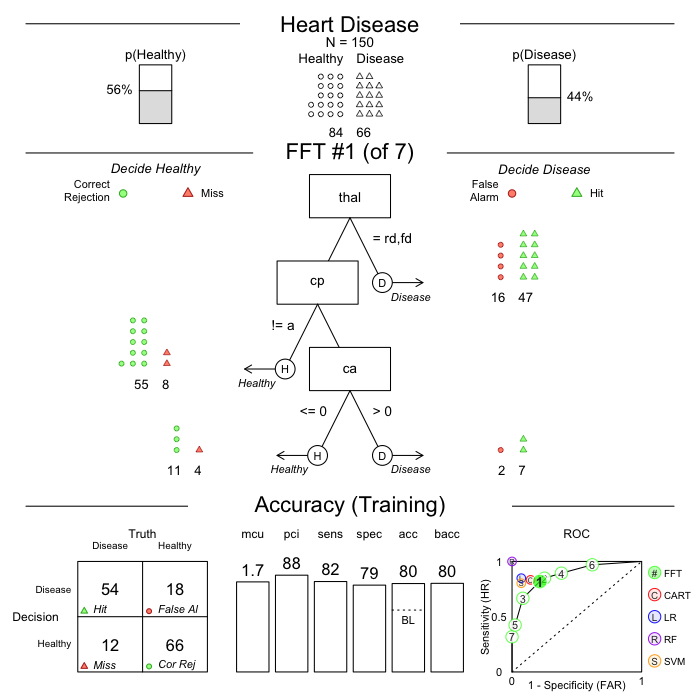## Specify sensitivity weights with `sens.w`

In some decision tasks, one might wish to weight the algorithm’s sensitivity differently than its specificity. For example, in cancer diagnosis, one might weight the algorithm’s sensitivity, the probability of detecting true cancer higher than the probability of correctly detecting true non-cancer. In other words, a miss might be more costly than a false-alarm. By default, `FFTrees` weights these two measures equally. To weight one measure more than the other, include a sensitivity weight `sens.w`:

``````# Breast cancer tree without specifying a sensitivity weight
breast.fft <- FFTrees(diagnosis ~.,
data = breastcancer)

plot(breast.fft)``````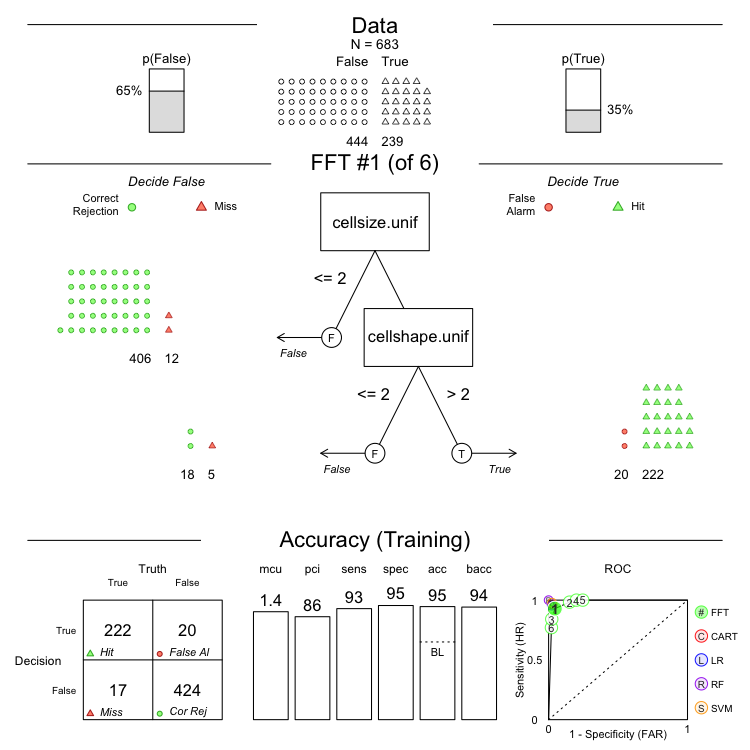This FFT had a sensitivity of 0.93 and a specificity of 0.95.

Now, let’s create a new FFTrees object and specify a desired sensitivity weight of .7:

``````# Breast cancer tree with a sensitivity weight of .7
breast2.fft <- FFTrees(diagnosis ~.,
data = breastcancer,
sens.w = .7)

plot(breast2.fft)``````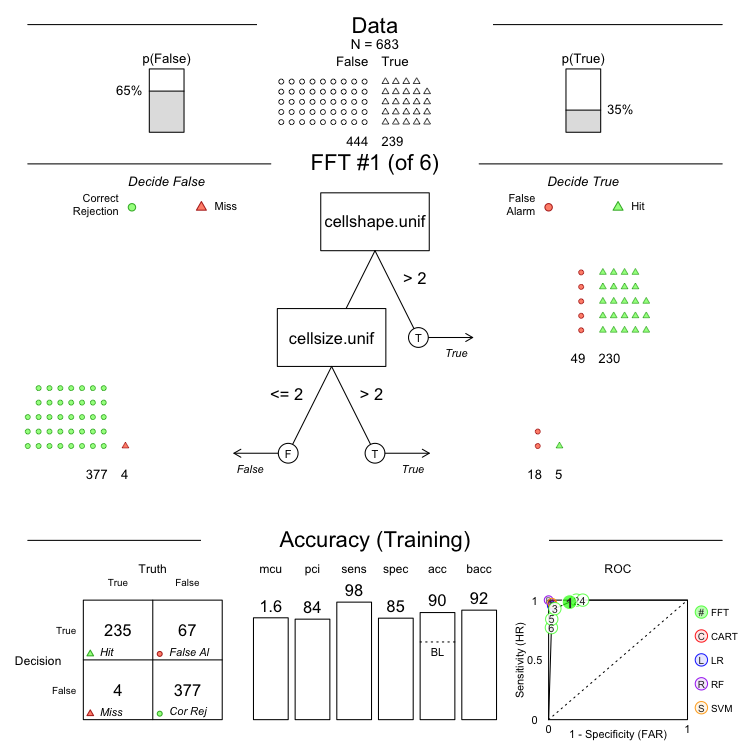The sensitivity for this FFT is a bit higher at 0.98, however, it came at a cost of a lower specificity of 0.85

• Specifying a `sens.w` value other than 0.5 does not (currently) actually affect how trees care constructed. Instead, it is used to select the tree with the highest weighted accuracy `wacc` score, where `wacc = sensitivity * sens.w + specificity * (1 - sens.w)` of all the trees contained in the `FFTrees` object.

## Define an FFT manually with `my.tree`

You can also define a specific FFT to apply to a dataset using the `my.tree` argument. To do so, specify the FFT as a sentence, making sure to spell the cue names correctly as the appear in the data. Specify sets of factor cues using brackets. In the example below, I’ll manually define an FFT using the sentence `"If chol > 300, predict True. If thal = {fd,rd}, predict False. Otherwise, predict True"`

``````# Define a tree manually using the my.tree argument
myheart.fft <- FFTrees(diagnosis ~.,
data = heartdisease,
my.tree = "If chol > 300, predict True. If thal = {fd,rd}, predict False. Otherwise, predict True")

# Here is the result
plot(myheart.fft,
main = "Specifying an FFT manually")``````